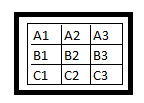## Thursday, November 12, 2015

### Determining the Determinant of a 3x3 matrix

Introduction
Determinant is a unique value representing a square matrix. It is useful in determining the inverse of a matrix. The determinant of a 1x1 matrix is the entry in the matrix itself whereas in the case of a 2x2 matrix, it is the upper left into lower right minus the upper right into the lower left. The determinant thus obtained is called the minor of the 2x2 matrix. Conventionally, the determinant of a higher order matrix is calculated by a recursive method with the help of the minors. Let us now see a method of rotations to find the determinant.
Let us consider the 2x2 matrix given on the left. Its determinant as per the rule given above is AD-BC.

However, look at the diagram given right. It can be explained as follows.
Rotation Method

Rotate left the second row to one position and then multiply the entries in the respective columns. Subtract the second column product from that of the first.This process can be extended into higher orders also.  This is done by carefully completing all the rotations depending on the number of rows. Let us see the case of a 3x3 matrix.

At first, rotate left the second row to one position and third row to two positions. The matrix thus obtained is given on the right.

Multiply the values in each column separately and then add the three products.
Thus we get A= (A1.B2.C3)+(A2.B3.C1)+(A3.B1.C2).

Now we rotate left the second row to one more position and the third row to two more positions. The matrix thus obtained is as follows.

Multiply the values in each column separately and then add the three products. Thus we get B=(A1.B3.C2)+(A2.B1.C3)+(A3.B2.C1).

The determinant of the 3x3 matrix is then
A-B = (A1.B2.C3+A2.B3.C1+A3.B1.C2)-( A1.B3.C2+A2.B1.C3+A3.B2.C1).

Cylindrical Rotation Method

This process is better understood if we can represent the matrix cylindrically. Consider a cylinder that has three horizontal sections which can be rotated freely with respect to a central vertical axis. Entries in the matrix are given on the exterior of the cylinder.

After the first set of two types of left rotations, the cylinder looks like what is on the left.

Multiplying the entries in the columns separately we get
A=(A1.B2.C3)+(A2.B3.C1)+(A3.B1.C2).

After the second set of two types of left rotations, the cylinder looks like what is on the right.

B = (A1.B3.C2)+(A2.B1.C3)+(A3.B2.C1).

The determinant of the 3x3 matrix is then
A-B = (A1.B2.C3+A2.B3.C1+A3.B1.C2)-( A1.B3.C2+A2.B1.C3+A3.B2.C1).

Palm Method

This can be visualized in yet another way also.
Let us use the three central fingers on the left palm to represent a 3x3 matrix.

Instead of the first set of rotations, multiply the entries from left as indicated by the dark lines, starting from the diagonal to get the value A.

To get the value B, we can multiply the entries from right starting from the antidiagonal. This can be done also by turning the palm and then multiplying the entries from left as given below from the diagonal.

Conclusion

An advantage of the above mentioned process is the elimination of the repeated use of plus(+) and minus(–) which is sometimes disturbing for beginners and non-Mathematics students. Can this method be extended to higher order matrices?

### Develop a skeleton for each day

One has to develop one's own habit of writing. One important  characteristic of habit is that one may not realize what one does in a hab...